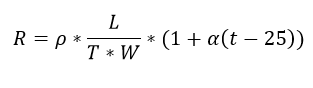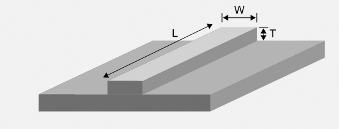# PCB Trace Resistance Calculator

This CalcTown calculator calculates the trace resistance of a printed circuit board.

The PCB Trace resistance is directly proportional to the length of the wire and inversely proportional to the area of the cross section of the wire. It also increases with the increase in temperature.

#### Result

ΩWhere,

ρ = resistivity of copper

α = temperature co-efficient of copper

T = trace thickness

W = trace width

L = trace length

t = temperature

We can pretty much neglect the DC resistance loss of a trace at high frequency. High frequency losses are dominated by skin effect and dielectric losses.

When using a trace’s resistance, it is important to take into account its large TCR, as well as processing variance. Also, the outside trace’s thickness can be significantly different than what the standard copper weight would imply. For example, to mitigate the TCR variation, one could use an appropriately positioned temperature sensor to monitor the trace’s temperature, and then compensate.